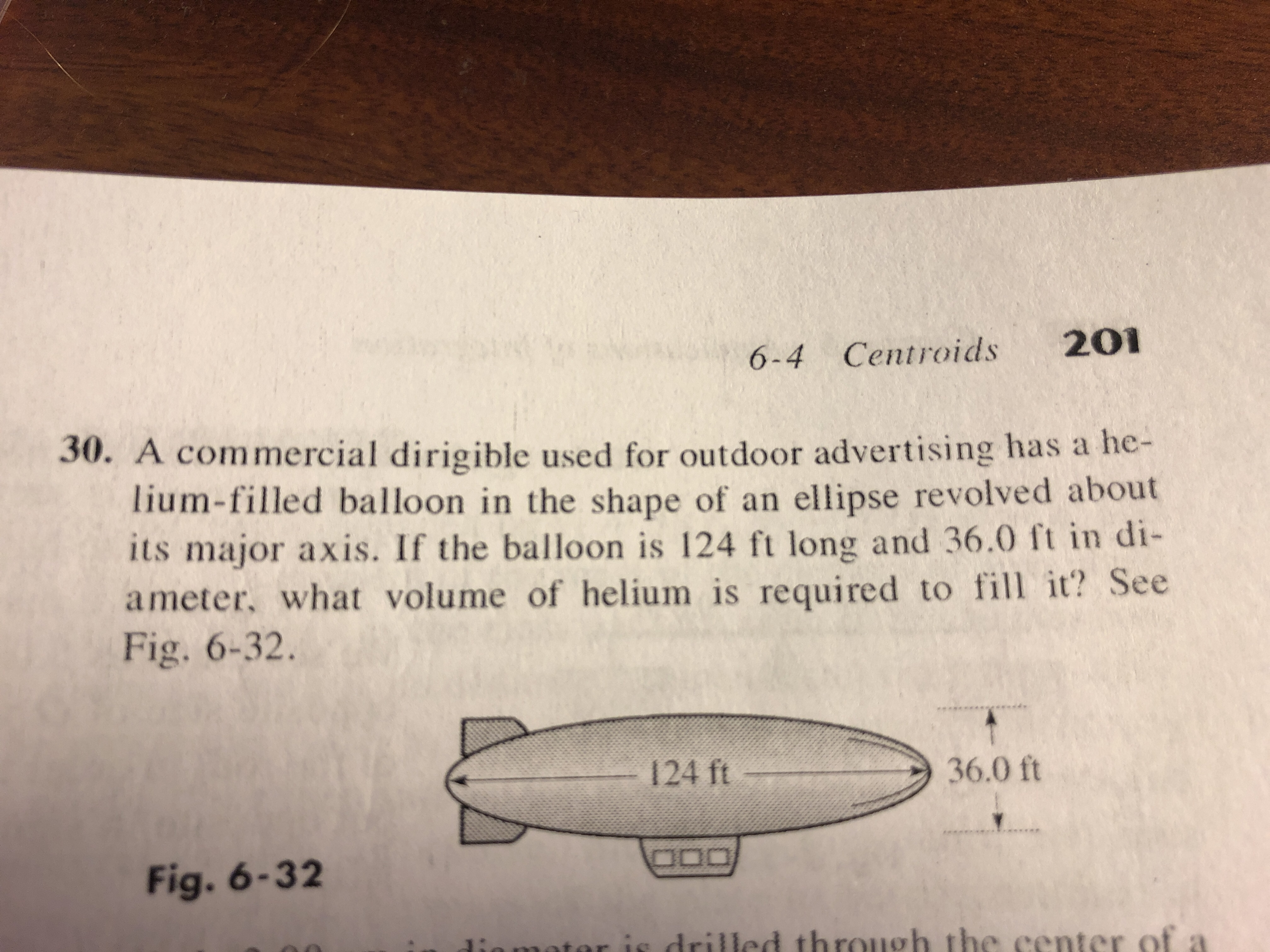# 6-4 Centroids 20130. A commercial dirigible used for outdoor advertising has a he-lium-filled balloon in the shape of an ellipse revolved aboutits major axis. If the balloon is 124 ft long and 36.0 ft in di-ameter, what volume of helium is required to t? SeeееFig. 6-32.36.0 ftFig. 6-32

Questionhelp_outlineImage Transcriptionclose6-4 Centroids 201 30. A commercial dirigible used for outdoor advertising has a he- lium-filled balloon in the shape of an ellipse revolved about its major axis. If the balloon is 124 ft long and 36.0 ft in di- ameter, what volume of helium is required to t? See ее Fig. 6-32. 36.0 ft Fig. 6-3 2 fullscreen
check_circleExpert Solution
Step 1

Please note that the volume of the ellipsoid is made up of aggregation of volume of various circular disc of radius y and thickness dx. One such disc is shown in the picutre on the white board.

Throughtout the answer to this question, we will make use of standard equation of ellipse that is x2 / a2 + y2 / b2 = 1 where length of major axis = 2a = 124 ft i.e. a = 124 / 2 = 62 and length of minor axis = 2b = 36 i.e. b = 36/2 = 18. We will do all the calulation using "a" and "b" substitute their values in the end.

Step 2

Consider the circular disc shown as hatched portion in the diagram above. It\'s radius = y. Hence, area, A = pi x (radius)2 = pi.y2; its thickness dx. Hence, its volume, dV = A.dx = pi.y2dx

From equation of ellipse, x2 / a2 + y2 / b2 = 1

Hence, y2 = b2 x (1 - x2 / a2)

Hence, volume of the disc, dV = pi.y2dx = pi. b2 x (1 - x2 / a2).dx

Range of integration will be x = -a to x = a. Since the shape is symmetrical, integration over x = - a to x= a will be equal to 2 x integration over x = 0 to x = a.

Step 3

Please see the white board f...

### Want to see the full answer?

See Solution

#### Want to see this answer and more?

Solutions are written by subject experts who are available 24/7. Questions are typically answered within 1 hour*

See Solution
*Response times may vary by subject and question
Tagged in

### Integration